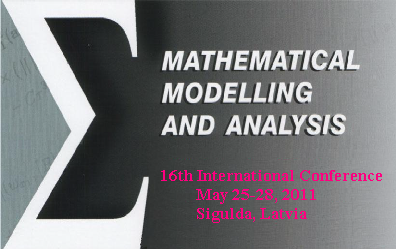16th International Conference on Mathematical Modelling and Analysis May 25-28, 2011, Sigulda, Latvia Conference ThemesThe themes of this conference include, but are not limited to: Analysis of numerical methods for solving problems of mathematical physics; Application of numerical methods to industrial and engineering problems; Parallel algorithms and parallel computing; Qualitative analysis and applications of ordinary and partial differntial equations as well difference equations problems; Financial mathematics and mathematics in economics; Scientific computation Mathematical modelling and analysis on the basis of fuzzy sets The scientific program includes invited plenary talks (40 min) and contributed talks (20 min). We also invite participants to organize mini-symposiums. The format for a mini-symposium might be a principal lecture and a number of communications of 15-20 minutes given by the other speakers. The mini-symposia will be scheduled in parallel sessions.   Last update: September 18, 2010 Designed by I.Bula